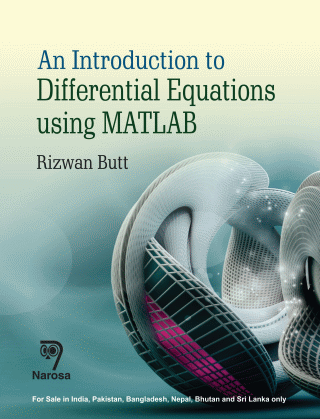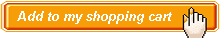Introduction to Differential Equations using MATLAB, An Authors:   Rizwan Butt ISBN: 978-81-8487-515-7 Publication Year:   2016 Pages:   592 Binding:   Paper Back About the book An Introduction to Differential Equations using MATLAB exploits the symbolic, numerical, and graphical capabilities of MATLAB to develop a thorough understanding of differential equations algorithms. This book provides the reader with numerous applications, m-files, and practical examples to problems. Balancing theoretical concepts with computational speed and accuracy, the book includes numerous short programs in MATLAB that can be used to solve problems involving first-and higher-order differential equations, Laplace transforms, linear systems of differential equations, numerical solutions of differential equations, computer graphics, and more. The author emphasizes the basic ideas of analytical and numerical techniques and the uses of modern mathematical software (MATLAB) rather than relying only on complex mathematical derivations to engineers, mathematician, computer scientists, and physicists or for use as a textbook in applied or computational courses. A CD-ROM with all the figures, codes, solutions, appendices, an introduction to MATLAB commands, and m-files for all of the programs is available on request. Key Features Table of content First-Order Differential Equations / Higher-Order Linear Differential Equations / Laplace Transforms / Linear Systems of Differential Equations / Series Solutions of Differential Equations / Numerical Solutions of Differential Equations / Partial Differential Equations / Index.Audience Under – Postgraduate Students & Researchers